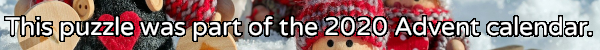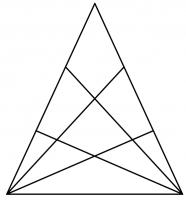mscroggs.co.uk
mscroggs.co.uksubscribe

# Puzzles

## 20 December18 can be written as the sum of 3 consecutive (strictly) positive integers: 5 + 6 + 7.
18 can also be written as the sum of 4 consecutive (strictly) positive integers: 3 + 4 + 5 + 6.
18 is in fact the smallest number that can be written as the sum of both 3 and 4 consecutive (strictly) positive integers.
Today's number is the smallest number that can be written as the sum of both 12 and 13 consecutive (strictly) positive integers.
Tags: numbers, sums

## 19 DecemberThe diagram to the right shows a triangle. Two of the sides of the triangle have been split into three pieces, with lines drawn from the opposite vertex. In total, the diagram now contains 27 triangles of any size.
Another triangle has two of its sides split into eight pieces, with lines drawn from the opposite vertex. How many triangles (of any size) would this create?

## 18 DecemberThe expansion of $$(x+y+z)^3$$ is
$$x^3 + y^3 + z^3 + 3x^2y + 3x^2z + 3xy^2 + 3y^2z + 3xz^2 + 3yz^2 + 6xyz.$$
This has 10 terms.
Today's number is the number of terms in the expansion of $$(x+y+z)^{26}$$.
Tags: algebra

## 17 DecemberPut the digits 1 to 9 (using each digit exactly once) in the boxes so that the sums and product are correct. Today's number is the product of the numbers in the red boxes.
 + + = 16 + + + + + = 8 + + + × × = 288 =11 =14 =20
Tags: numbers, grids

## 16 DecemberSolve the crossnumber to find today's number. No number starts with 0.

## 15 DecemberWhen talking to someone about this Advent calendar, you told them that the combination of XMAS and MATHS is GREAT. They were American, so asked you if the combination of XMAS and MATH is great; you said SURE. You asked them their name; they said SAM.
Each of the letters E, X, M, A, T, H, S, R, U, and G stands for a different digit 0 to 9. The following sums are correct:
Today's number is SAM. To help you get started, the letter T represents 4.

## 14 DecemberThe numbers 33, 404 and 311 contain duplicate digits. The numbers 120, 15 and 312 do not.
How many numbers between 10 and 999 (inclusive) contain no duplicate digits?

## 13 DecemberThere are 6 ways to split the sequence of the numbers 1 to 5 into three shorter sequences:
• 1 and 2 and 3, 4, 5
• 1 and 2, 3 and 4, 5
• 1 and 2, 3, 4 and 5
• 1, 2 and 3 and 4, 5
• 1, 2 and 3, 4 and 5
• 1, 2, 3 and 4 and 5
Today's number is the number of ways to split the sequence of the numbers 1 to 10 into five shorter sequences.

## Archive

Show me a random puzzle
▼ show ▼